American Journal of Applied Mathematics
Volume 4, Issue 6, December 2016, Pages: 271-276

Solving Porous Medium Equation Using Aboodh Transform Homotopy Perturbation Method

Khalid Suliman Aboodh1, 2

1Department of Mathematics, Faculty of Science &Technology, Omdurman Islamic University, Khartoum, Sudan

2Department of Mathematics, Faculty of Science & Arts, University of Bisha, Bisha, KSA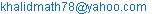Khalid Suliman Aboodh. Solving Porous Medium Equation Using Aboodh Transform Homotopy Perturbation Method. American Journal of Applied Mathematics. Vol. 4, No. 6, 2016, pp. 272-277.doi: 10.11648/j.ajam.20160406.12

Received: September 18, 2016; Accepted: October 10, 2016; Published: November 1, 2016

Abstract: In this paper, a new technique has been used forsolving the porous medium equation. This method is named by Aboodh transform which is a combination of the new integral transform "Aboodh transform" and the homotopy perturbation method. The nonlinear termin the porous medium equationscan be easily handled by homotopy perturbation method and some cases of these Equations are solved as examples to illustrate ability and reliability of mixture of Aboodh transform and homotopy perturbation method. The results reveal that the combination of Aboodh transform and homotopy perturbation methodis quite capable, practically well appropriate for usingsuch theproblems. Also, this method is found that a better alternative method to some existing techniques for such realistic problems.

Keywords: Aboodh Transform Homotopy Perturbation Method, Non Linear Partial Differential Equation, Porous Medium Equation

Contents

1. Introduction

Many of the physical phenomena and processes in variousfields of engineering and science are governed by partialdifferential equations. The nonlinear heat equationdescribing various physical phenomena called the porousmedium equation. The porous medium equation  is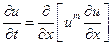(1)

Where m is a rational number, is a prominent example of nonlinear partial differential equation. In the particular case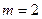it leads to Boussinesqequation. This equation is one of the simplest examples of nonlinear evolution equation of parabolic type. It appears in the description of different natural phenomena, and its theory and properties depart strongly from the heat equation,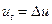, its most famous relative. There are a number of physical applications where this simple equation appears in a natural way, mainly to describe processes involving fluid flow, heat transfer or diffusion. May be the best known of them is the description of the flow of an isentropic gas through a porous medium, modelled independently by Leibenzon and Musk at around 1930. An earlier application is found in the study of groundwater infiltration by Boussisnesq in 1903. Another important application refers to heat radiation in plasmas, developed by Zel'dovich and co-workers around 1950. Indeed, this application was at the base of the rigorous mathematical development of the theory. Other applications have been proposed in mathematical biology, spread of viscous fluids, boundary layer theory, and other fields. With the rapid development of nonlinear science, there has appeared ever -increasing interest of scientists and engineers in the analytical asymptotic techniques for nonlinear problems such as solid state physics, plasma physics, fluid mechanics and applied sciences.Several techniques including the Adomain’s decomposition method, the Variational iteration method, the weighted finite difference method, the Laplace decomposition method and the Variational iteration decomposition method have their own limitation like the calculation of Adomain’s polynomial s and the Lagrange’s multipliers. The results obtained by these methods are divergent in most cases and which results in causing a lot of chaos. To overcome these difficulties and drawbacks such new techniques are introduced for finding the approximate results.Motivated and inspired by the ongoing research in these areas, we consider a new method, which is called the Homotopy perturbation transform method (HPTM). The suggested HPTM provides the solution in a rapid convergent series which may leads the solution in a closed form. The advantage of this method is its capability of combining of two powerful methods for obtaining exact solution for nonlinear equations. The use of He’s polynomials in the nonlinear term was first introduced by Gorbhani. It is worth mentioning that the HPTM is applied without any discretization or restrictive assumptions or transformations and free from round -off errors.

These reasons support the interest of its study both for the mathematician and the scientist.

In recent years, many research workers have paid attention to find the solution of nonlinear differential equations by using various methods. Among these are the Adomian decomposition method [Hashim, Noorani, Ahmed. Bakar, Ismail and Zakaria, (2006)], the tanh method, the homotopyperturbation method [Sweilam, Khader (2009), Sharma and Giriraj Methi (2011), Jafari, Aminataei (2010), (2011)], thedifferential transform method [(2008)], homotopy perturbation transform method and the variational iteration method. Various ways have been proposed recently to deal with these nonlinearities; one of these combinations is Aboodh transform and homotopy perturbation method.

Aboodh transform is a useful technique for solving linear differential equations but this transform is totally incapable of handling nonlinear equations  because of the difficulties that are caused by the nonlinear terms. This paper is using homotopy perturbation method to decomposethe nonlinear term, so that the solution can be obtained by iteration procedure. This means that we can use both Aboodh transform and homotopy perturbation methods to solve many nonlinear problems. The main aim of this paper is to consider the effectiveness of the Aboodh transform homotopy perturbation method in solving nonlinear porous medium equations. This method provides the solution in a rapidconvergent series which may leads the solution in a closed form. The fact that the proposed technique solves nonlinear problems without using so-called Adomian's polynomials is a clear advantage of this algorithm over the decomposition method.

2. Aboodh Transform Homotopy Perturbation Method 

Consider a general nonlinear non-homogenous partial differential equation with initial conditions of the form: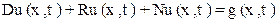(2)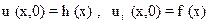Where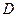is linear differential operator of order two,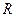is linear differential operator of less order than,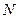is the general nonlinear differential operator and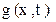is the source term.

Taking Aboodh transform on both sides of equation (2), to get: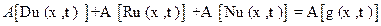(3)

Using the differentiation property of Aboodh transforms and above initial conditions, we have: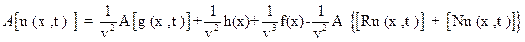(4)

Appling the inverse Aboodh transform on both sides of equation (4), to find: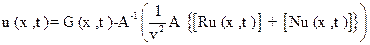(5)

Where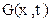represents the term arising from the source term and the prescribed initial conditions. So that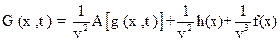Now, we apply the homotopy perturbation method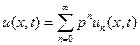(6)

And the nonlinear term can be decomposed as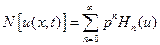(7)

Where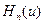are given by: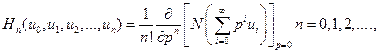(8)

Substituting equations (6) and (7) in equation (5), we get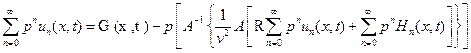(9)

This is the coupling of the Aboodh transform and the homotopy perturbation method. Comparing the coefficient of like powers of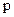, the following approximations are obtained.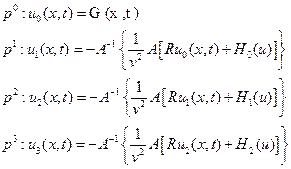(10)

The best approximations for the solutions are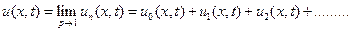(11)

3. Applications

Now, we consider in this section the effectiveness of the Aboodh transform homotopy-perturbation method to obtain the exact and approximate analytical solution of the porous medium equations.

Example 3.1

Let us take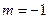in equation (1), we get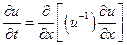(12)

With initial condition as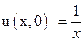. Exact solution  of this equation is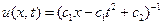With the values of arbitrary constants taken as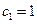and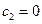solution becomes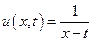we can find solution by applying Aboodh transform on both side of equation (12) subject to the initial condition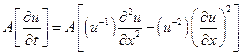(13)

This can be written as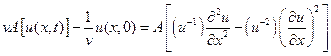(14)

On applying the above specified initial condition, we get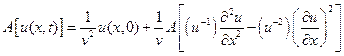(15)

Taking inverse Aboodh transformon both sides of Eq. (15), we get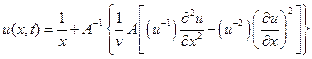(16)

Now, we apply the homotopy perturbation method(17)

And the nonlinear term can be decomposed as(18)

Using Eqs.(17)-(18) into Eq.(16) we get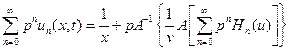(19)

Whereare He'spolynomials. The first two components of He's polynomials are given by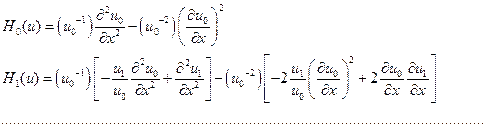Comparing the coefficient of various power of p in (19), we get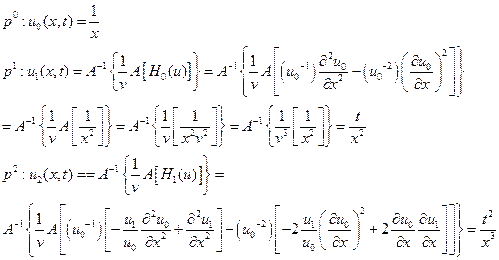Proceeding in similar manner we can obtain further values,

Substituting above values in equation (10), we get solution in the form of a series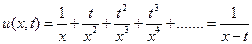(20)

This is the solution of (12) and which is exactly the exact solution given above.

Example 3.2

Let us take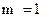in equation (1), we get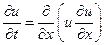(21)

with initial condition as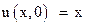Exact solution  of this equation is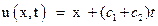with the values of arbitrary constants taken asandsolution becomes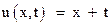.

We can find solution by applying Aboodh transform on both

side of equation (21)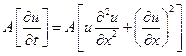(22)

This can be written as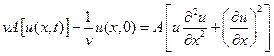(23)

On applying the above specified initial condition, we get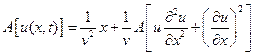(24)

Taking inverse Aboodh transform on both sides of Eq. (24), we get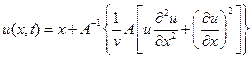(25)

Now, we apply the homotopy perturbation method,(26)

And the nonlinear term can be decomposed as(27)

Invoking Eqs. (26)- (27) into Eq. (25), we get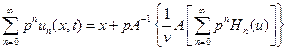(28)

Whereare He's polynomials. The first two components of He's polynomials are given byComparing the coefficient of various power of p in (28), we get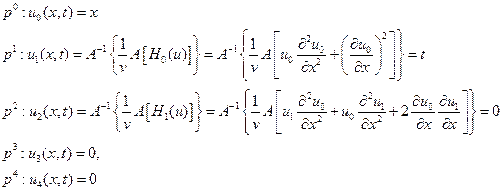And so on we will found that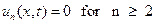Substituting above values in equation (11) we get solution in the form of a series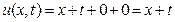(29)

This is the solution of (21) and which is exactly the exact solution given above.

Example 3.3

Let us take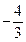in equation (1), we get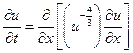(30)

With initial condition as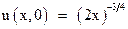using afore said method, we have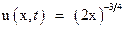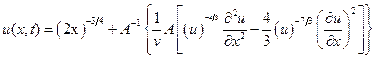(31)

Now we apply the homotopy perturbation method,(32)

And the nonlinear term can be decomposed as(33)

Invoking Eqs. (32)- (33) into Eq. (31), we get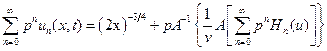(34)

Whereare He's polynomials. The first two components of He's polynomials are given byComparing the coefficient of various power of p in (34), we get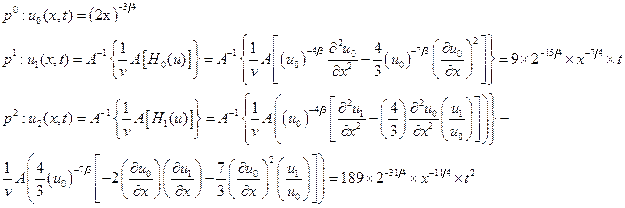On substituting these terms in equation (10), we obtained the solution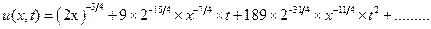This result can be verified through substitution.

4. Conclusion

The main goal of this paper is to show the applicability of the mixture of new integral transform "Aboodh transform" with the homotopy perturbation method to construct an analytical solution for porous medium equation. This combination of two methods successfully worked to give very reliable and exact solutions to the equation. This method provides an analytical approximation in a rapidly convergent sequence with in exclusive manner computed terms. Its rapid convergence shows that the method is Trustworthy and introduces a significant improvement in solving nonlinear partial differential equations over existing methods. Finally it's worthwhile to mention that the proposed method can be applied to other linear and nonlinear partial differential equations arising in mathematical physics and application in different field of sciences. This aim task in future.

References

1. A. D. Polyanin, V. F. Zaitsev (2004), Handbook of Nonlinear Partial Differential Equations, Chapman and Hall/CRC Press, Boca Raton, 2004.
2. Mishra D, Pradhan V. H., Mehta M. N. (2012), Solution of Porous Medium Equation by Homotopy Perturbation Transform Method, International Journal of Engineering Research and Applications, Vol. 2 Issue 3, pp 2041-2046.
3. Juan Luis Vazquez (2007), The Porous Medium Equation Mathematical Theory, Oxford Science Publication, Clarenden Press, pp 1-28.
4. Khalid Suliman Aboodh, Homotopy Perturbation Method and Aboodh Transform for Solving Nonlinear Partial Differential Equations, Aboodh Transform for Solving Nonlinear Partial Differential Equations, Theory of Approximationand Applications ISSN: 2326-9790 (Print); February 2016.
5. Tarig M. Elzaki and Salih M. Elzaki (2011), Applications of New Transform "ELzaki Transform" to Partial Differential Equations, Global Journal of Pure and Applied Mathematics, Vol. 7, No. 1, pp 65-70.
6. Khalid Suliman Aboodh, The New Integral Transform ''Aboodh Transform'' Global Journal of Pure and Applied Mathematics ISSN 0973-1768 Volume 9, Number 1 (2013), pp. 35-43.
7. Tarig M. Elzaki, Salih M. Elzaki and Elsayed A. Elnour (2012), On the New Integral Transform "ELzaki Transform" Fundamental Properties Investigations and Applications, Global Journal of Mathematical Sciences: Theory and Practical, Vol. 4, No. 1, pp 1-13.
8. Khalid Suliman Aboodh, Application of New Transform "Aboodh Transform" to Partial Differential Equations ''Global Journal of Pure and Applied Mathematics ISSN 0973-1768 Volume 10, Number 2 (2014), pp. 249-254
9. Tarig M. ElzakiandSalih M. Elzaki, Applications of New Trans form "ELzaki Transform" to Partial Differential Equations, Global Journal of Pure and Applied Mathematics, (7) 1, 2011, pp 65-70.
10. He J. Homotopy-perturbation method for solving boundary value problem, Phys Lett A, 350 (2006), 87-88.
11. Prem Kiran and G. Bhadane, ELzaki Transform Homotopy-perturbation method for solving Porous equation, International Journal of Research in Engineering and Technology, Volume: 02 Issue: 12- Dec-2013.
12. Khalid Suliman Aboodh, Solving Fourth Order Parabolic PDE with Variable Coefficients Using Aboodh Transform Homotopy Perturbation Method, Pure and Applied Mathematics Journal 2015; 4 (5): 219-224.
13. Tarig M. Elzaki and Eman M. A. Hilal (2012), Homotopy Perturbation and ELzaki Transform for solving Nonlinear Partial Differential equations, Mathematical Theory and Modeling, Vol. 2, No. 3, pp 33-42.

 Contents 1. 2. 3. 4.
Article ToolsAbstractPDF(171K)